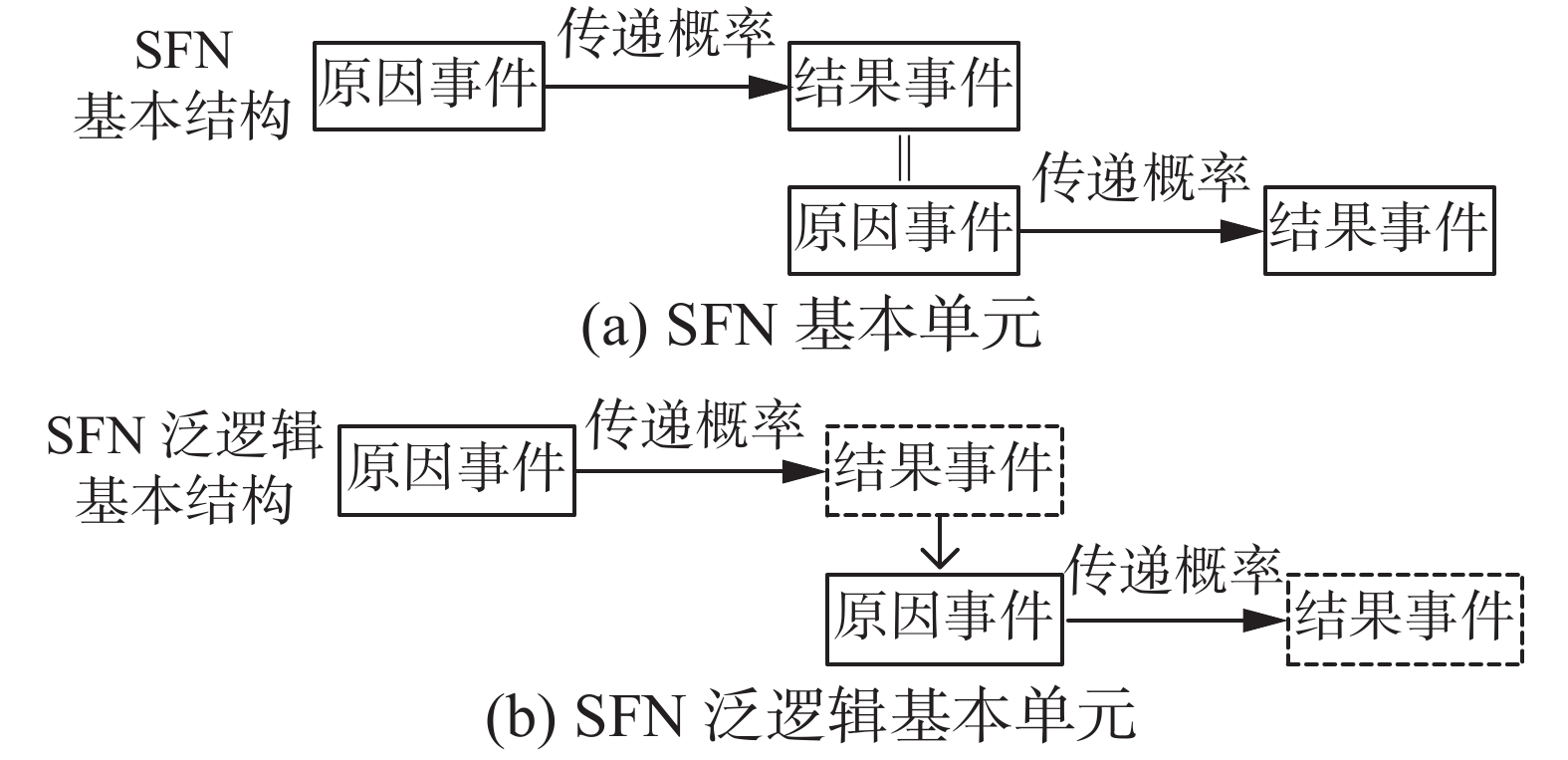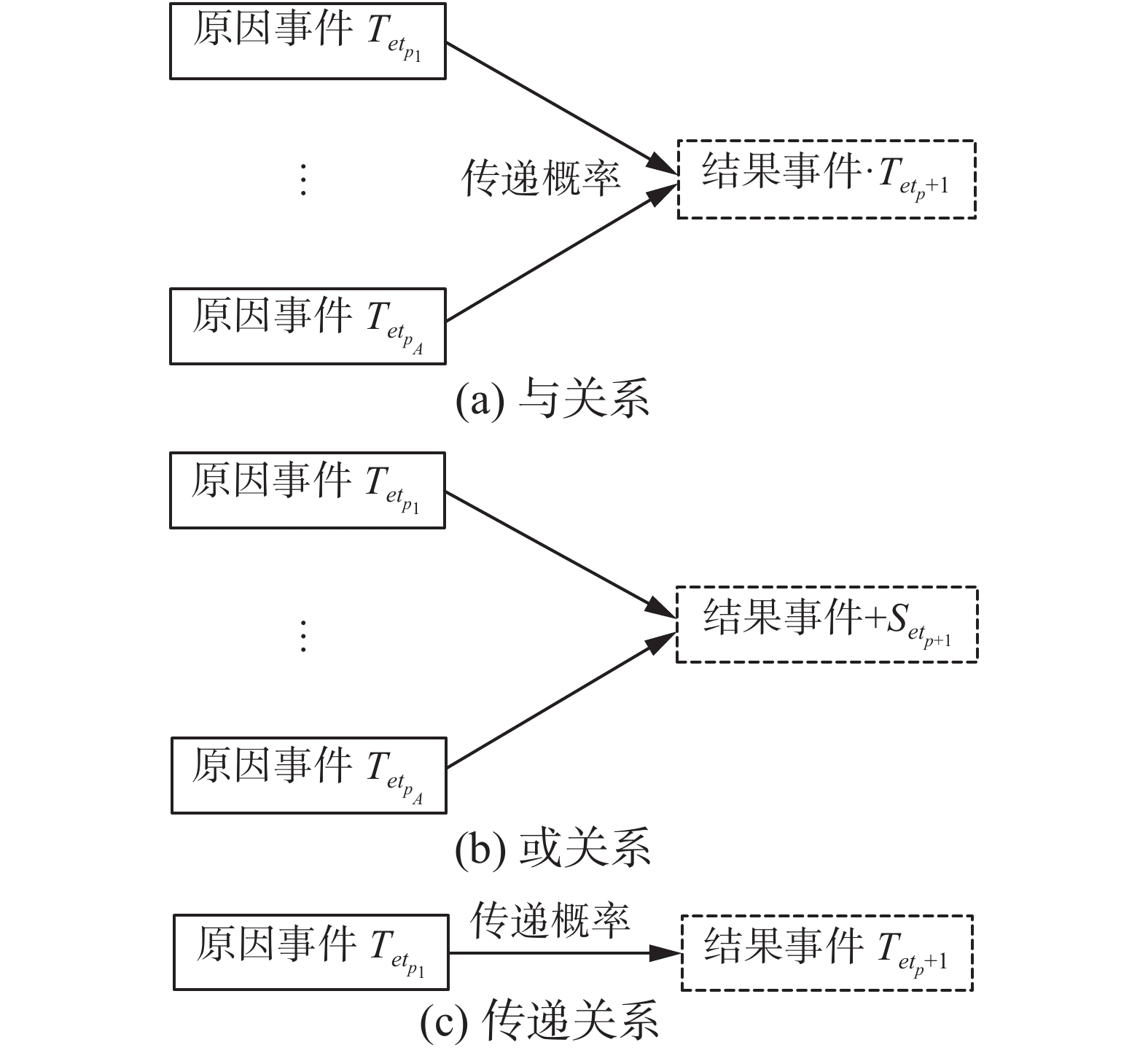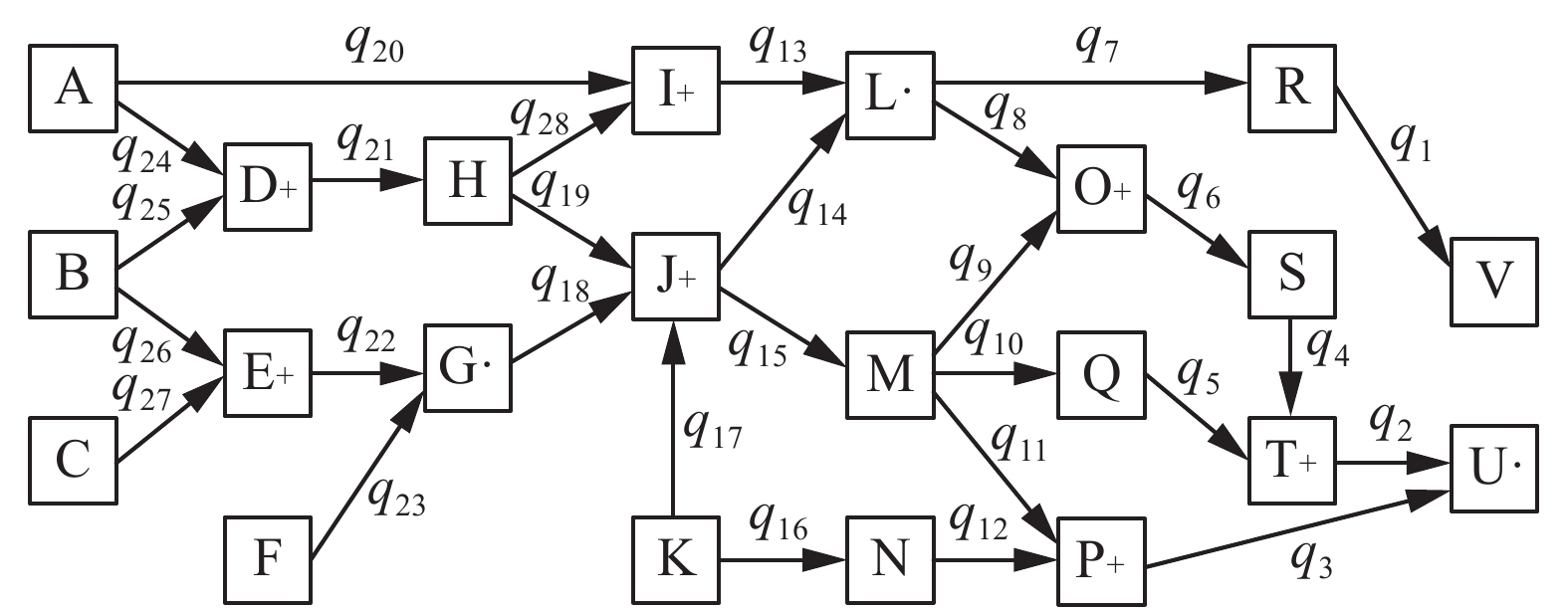﻿ 空间故障网络的柔性逻辑描述
«上一篇文章快速检索 高级检索

 智能系统学报2021, Vol. 16Issue (3): 552-559  DOI: 10.11992/tis.2020030290

### 引用本文CUI Tiejun, LI Shasha. Flexible logic description of space fault network[J]. CAAI Transactions on Intelligent Systems, 2021, 16(3): 552-559. DOI: 10.11992/tis.202003029.### 文章历史

1. 辽宁工程技术大学 安全科学与工程学院，辽宁 阜新 123000;
2. 大连交通大学 辽宁省隧道与地下结构工程技术研究中心，辽宁 大连 116028;
3. 辽宁工程技术大学 工商管理学院，辽宁 葫芦岛 125105

Flexible logic description of space fault network
CUI Tiejun 1,2, LI Shasha 3
1. College of Safety Science and Engineering, Liaoning Technical University, Fuxin 123000, China;
2. Tunnel & Underground Structure Engineering Center of Liaoning, Dalian Jiaotong University, Dalian 116028, China;
Abstract: Flexible logic is proposed in this paper to describe and transform the space fault network (SFN) and to describe the system fault evolution process (SFEP) with information uncertainty and evolution characteristics to overcome the factors, data, and evolution uncertainty. The present report discusses the problems of SFN and the possibility of using flexible logic to solve these problems. The use of flexible logic to describe SFN should be based on the basic unit, event occurrence relationship, and SFN structure. Flexible logical representations of the and, or and transfer relationship in SFN are given. Further, under the condition of flexible logic, the relationship set of SFN is given, and the flexible logic representation of the target event state of SFN is obtained. The results show the flexible logical relationship and uncertainty among events, factors, and evolution processes in SFEP and provide a new methodology and theoretical basis for the intelligent research of SFEP.
Key words: intelligent science    safety science    safety system engineering    space fault network    flexible logic    system fault evolution process    factor influence    uncertainty

SFEP[1-7]存在于各行各业，无论是自然系统灾害还是人工系统故障都是系统功能性下降或故障性升高的结果。SFEP中蕴含了一系列事件，受到多种因素影响，事件间也存在不同的逻辑关系，因此SFEP的事先分析极其困难。因为SFEP中各种事件和它们之间的逻辑关系，加之不同因素的影响，使得SFEP存在多样性。事先分析只能得到各种SFEP的可能性，只有发生后才能确定是哪一种SFEP。那么如何智能化地事先分析SFEP成为关键问题。

1 空间故障网络

SFN是空间故障树理论[1-7,35-46](space fault tree, SFT)的第三研究阶段。SFT包括SFT基础理论[35-39]、智能化SFT[40-46]、SFN[1-7]、系统运动空间与系统映射论。SFN用于描述SFEP。SFEP代表了系统性能变化过程中各种事件、各种逻辑关系和各种因素的联系。SFN通过网络拓扑形式表示SFEP。

SFN由点和线组成。点表示SFEP的事件，线表示SFEP的演化途径。在SFEP中多个原因事件可能存在不同逻辑关系导致结果事件，最典型的是同时发生的与关系和之一发生的或关系。在理论上使用SFN描述SFEP是适合的，但在实际过程中存在问题。其中最重要的就是不确定性和演化特征。

2 柔性逻辑

3 柔性逻辑描述

SFN描述SFEP，SFEP中的事件、传递概率和事件发生关系分别对应SFN中的节点、连接和因果逻辑。但为了说明方便，在论述SFN时也使用事件、传递概率和事件发生关系。SFN的柔性逻辑描述要从几个方面来讨论，包括：SFN最基本单元的柔性逻辑描述、事件发生关系的柔性逻辑描述、SFN结构的柔性逻辑描述。

3.1 SFN最基本单元的柔性逻辑描述

SFN的基本单元：原因事件→传递概率→结果事件，将结果事件作为下一个单元的原因事件继续通过传递概率指向下一个结果事件，如图1(a)所示。整个过程起始于边缘事件(导致SFEP的最基本事件)，终止于最终事件(SFEP结束的事件或过程中关心的事件)。将基本单元进一步分解，原因事件与传递概率组成SFN柔性逻辑基本单元。所得结果作为原因事件与下一个传递概率形成柔性逻辑基本单元，如图1(b)所示。因此SFN的基本单元从原来的三元组变为两元组。该变化原因在于柔性逻辑的逻辑运算完整簇形式[29-30]。柔性逻辑运算完整簇被规定为一个二元算子，两个输入一个输出，是为了简化逻辑表达，也因为任何逻辑运算都可拆分为二元运算。Download: 图 1 SFN的基本单元 Fig. 1 Basic unit of SFN

 \begin{aligned} &{T_{et_p}}({e_p},t{p_q},k,h,\beta ) = \\ &{(\max (0,2\beta {e_p^{nm}} + 2(1 - \beta )t{p_q^{nm}} - 1))^{\frac{1}{{nm}}}} \end{aligned} (1)

3.2 事件发生关系的柔性逻辑描述Download: 图 2 柔性逻辑表示的SFN事件发生关系 Fig. 2 SFN event occurrence relationship represented by flexible logic

 \left\{ {\begin{aligned} {T_{et_{p} + 1}}= T(T( \cdots (T({T_{et_{p_1}}},{T_{et_{p_2}}}),{T_{et_{p_3}}}) \cdots ),{T_{et_{p_A}}})\\ \quad T({T_{et_{p_1}}},{T_{et_{p_2}}}) = T({T_{et_{p_1}}},{T_{et_{p_2}}},k,h,\beta ) =\quad\;\;\\ {(\max (0,2\beta {T_{et_{p_1}}}^{nm} + 2(1 - \beta ){T_{et_{p_2}}}^{nm} - 1))^{\frac{1}{{nm}}}}\;\;\; \end{aligned}} \right. (2)

 \left\{{\begin{aligned} &{S_{et_{p} + 1}} = S(S( \cdots (S({T_{et_{p_1}}},{T_{et{p_2}}}),{T_{et_{p_3}}}) \cdots ),{T_{et_{p_A}}})\\ &S({T_{et_{p_1}}},{T_{et_{p_2}}}) = S({T_{et_{p_1}}},{T_{et_{p_2}}},k,h,\beta ) =\\ & \quad\quad(1 - (\max (0,2\beta {(1 - {T_{et_{p_1}}^n})^m} + \quad\quad \\ &\quad\quad2(1 - \beta ){(1 - {T_{et_{p_2}}^n})^m} - 1))^{\frac{1}{m}})^{\frac{1}{n}} \quad \quad\\ \end{aligned}} \right. (3)

 \left\{ {\begin{aligned} &{{T_{et_{p} + 1}} = {T_{et_{p_1}}}} \\ &{T_{et_p}}({e_p},t_{p_q},k,h,\beta ) = \\ &\quad{(\max (0,2{\beta_{{e_p}}^{nm}} + 2(1 - \beta )t{p_q^{nm}} - 1))}^{\frac{1}{nm}} \end{aligned}} \right. (4)
3.3 SFN结构的柔性逻辑描述

 $\begin{array}{l} {\rm{RS}} = \{ {e_{{\rm{CE}}}} \to {e_{{\rm{RE}}}}|{e_{{\rm{RE}}}} = {S_{et_{p} + 1}}{\rm{,or}};{e_{{\rm{RE}}}} = {T_{et_{p} + 1}}{\rm{,and;}} \\ {e_{{\rm{RE}}}} = {T_{et_{p_1}}}{\rm{,trans;}}{e_{{\rm{CE}}}}{\rm{, }}{e_{{\rm{RE}}}} \in {\rm{\{ 0,1\} }}\} \\ \end{array}$ (5)

4 SFN的柔性逻辑描述举例Download: 图 3 空间故障网络 Fig. 3 Space fault network(SFN)

 $\begin{split} {e_v} =& {T_{et_{p}}}({T_{et_{p}}}({T_{et_{p}}}({T_{et_{p}}}({S_{et_{p}}}({T_{et_{p}}}({e_{\rm{A}}},{q_{20}}),\\ &{T_{et_{p}}}({T_{et_{p}}}({S_{et_{p}}}({T_{et_{p}}}({e_{\rm{A}}},{q_{24}}),{T_{et_{p}}}({e_{\rm{B}}},{q_{25}})),{q_{21}}),{q_{28}})),{q_{13}}),\\ &{T_{et_{p}}}({S_{et_{p}}}({S_{et_{p}}}({T_{et_{p}}}({T_{et_{p}}}({S_{et_{p}}}({T_{et_{p}}}({e_{\rm{A}}},{q_{24}}),\\ &{T_{et_{p}}}({e_{\rm{B}}},{q_{25}})),{q_{21}}),{q_{19}}),{T_{et_{p}}}({T_{et_{p}}}({T_{et_{p}}}({S_{et_{p}}}({T_{et_{p}}}({e_{\rm{B}}},{q_{26}}),\\ &{T_{et_{p}}}({e_{\rm{C}}},{q_{27}})),{q_{22}}),{T_{et_{p}}}({e_{\rm{F}}},{q_{23}})),{q_{18}})),\\ &{T_{et_{p}}}({e_{\rm{K}}},{q_{17}})),{q_{14}})),{q_7}),{q_1}) \end{split}$ (6)

5 结束语

1)论述了使用SFN描述和研究SFEP存在的问题。由于SFEP自身特点，对其进行描述和研究存在的问题主要包括因素的不确定性、数据的不确定性和SFEP本身的逻辑关系，及它们出现的原因。

2)论述了柔性逻辑情况。给出了泛逻辑学的基本目的和基本形式，论述了使用柔性逻辑描述和研究SFEP的优势，从而对SFN进行了柔性逻辑表示。

3)论述了SFN的柔性逻辑具体描述方法。根据SFEP和SFN特征，给出了柔性逻辑描述方法，包括SFN最基本单元描述、事件发生关系描述、SFN结构描述。研究了SFN中与、或和传递关系转化为柔性逻辑关系的方式。将原因事件和传递概率设置为SFN柔性逻辑基本单元，其结果作为本次结果事件状态和下次原因事件状态。再结合SFN柔性逻辑关系组，即可得到SFN最终事件状态。柔性逻辑的20种形式都可进行类似转化，在丰富SFN事件发生逻辑关系的同时，也使SFN具备了使用泛逻辑方法论的基础。

4)使用实例SFN得到了柔性逻辑最终事件状态表达式。这种表达式可以表达SFEP中各事件、各因素和演化过程之间的柔性逻辑关系，并表示它们的不确定性。

  崔铁军, 李莎莎, 朱宝岩. 空间故障网络及其与空间故障树的转换[J]. 计算机应用研究, 2019, 36(8): 2400-2403. CUI Tiejun, LI Shasha, ZHU Baoyan. Construction space fault network and recognition network structure characteristic[J]. Application research of computers, 2019, 36(8): 2400-2403. (0)  崔铁军, 李莎莎, 朱宝岩. 含有单向环的多向环网络结构及其故障概率计算[J]. 中国安全科学学报, 2018, 28(7): 19-24. CUI Tiejun, LI Shasha, ZHU Baoyan. Multidirectional ring network structure with one-way ring and its fault probability calculation[J]. China safety science journal, 2018, 28(7): 19-24. (0)  CUI Tiejun, LI Shasha. Research on complex structures in space fault network for fault data mining in system fault evolution process[J]. IEEE access, 2019, 7: 121881-121896. DOI:10.1109/ACCESS.2019.2937641 (0)  崔铁军, 李莎莎. 空间故障树与空间故障网络理论综述[J]. 安全与环境学报, 2019, 19(2): 399-405. CUI Tiejun, LI Shasha. Revision of the space fault tree and the space fault network system[J]. Journal of safety and environment, 2019, 19(2): 399-405. (0)  崔铁军. 系统故障演化过程描述方法研究[J]. 计算机应用研究, 2020, 37(10): 3006-3009. CUI Tiejun. Research on description method of system fault evolution process[J]. Application research of computers, 2020, 37(10): 3006-3009. (0)  CUI Tiejun, LI Shasha. Research on basic theory of space fault network and system fault evolution process[J]. Neural computing and applications, 2020, 32(6): 1725-1744. DOI:10.1007/s00521-019-04247-0 (0)  CUI Tiejun, LI Shasha. Deep learning of system reliability under multi-factor influence based on space fault tree[J]. Neural computing and applications, 2019, 31(9): 4761-4776. DOI:10.1007/s00521-018-3416-2 (0)  QIU Xiaohong, HU Yuting, LI Bo. Sequential fault diagnosis using an inertial velocity differential evolution algorithm[J]. International journal of automation and computing, 2019, 16(3): 389-397. DOI:10.1007/s11633-016-1008-0 (0)  GUEDES J J, CASTOLDI M F, GOEDTEL A, et al. Differential evolution applied to line-connected induction motors stator fault identification[J]. Soft computing, 2019, 23(21): 11217-11226. DOI:10.1007/s00500-018-03674-w (0)  万蔚, 黄雨晨, 王振华, 等. 突发状况下的道路网络故障演化分析——以通州市区道路网络为例[J]. 重庆交通大学学报(自然科学版), 2019, 38(1): 14-20, 40. WAN Wei, HUANG Yuchen, WANG Zhenhua, et al. Evolution analysis of road network faults under emergency conditions—taking Tongzhou urban road network as an example[J]. Journal of Chongqing Jiaotong University (natural sciences), 2019, 38(1): 14-20, 40. DOI:10.3969/j.issn.1674-0696.2019.01.03 (0)  张保山, 张琳, 张搏, 等. 基于故障风险标尺的复杂装备健康状态分类模型[J]. 系统工程与电子技术, 2020, 42(2): 489-496. ZHANG Baoshan, ZHANG Lin, ZHANG Bo, et al. Equipment health classification model based on failure risk scale[J]. Systems engineering and electronics, 2020, 42(2): 489-496. DOI:10.3969/j.issn.1001-506X.2020.02.31 (0)  范海东, 王玥, 李清毅, 等. 基于稀疏故障演化判别分析的故障根源追溯[J]. 控制工程, 2019, 26(7): 1239-1244. FAN Haidong, WANG Yue, LI Qingyi, et al. Sparse fault degradation oriented fisher discriminant analysis based fault trace[J]. Control engineering of China, 2019, 26(7): 1239-1244. (0)  于洋洋. 面向文本数据的故障模型挖掘技术研究[D]. 青岛: 青岛科技大学, 2019. YU Yangyang. Research on text-oriented fault model mining technology[D]. Qingdao: Qingdao University of Science & Technology, 2019. (0)  曾振城. 海底电缆短路故障演化机理和诊断方法研究[D]. 厦门: 集美大学, 2019. ZENG Zhencheng. Research on evolution mechanism and diagnosis method of submarine cable short circuit fault[D]. Xiamen: Jimei University, 2019. (0)  李文博. 交直流混联系统连锁故障搜索方法及应用研究[D]. 济南: 山东大学, 2019. LI Wenbo. Research on search method and its application for cascading failures in AC/DC hybrid system[D]. Ji’nan: Shandong University, 2019. (0)  张冕. 行星齿轮箱关键零部件故障诊断[D]. 成都: 电子科技大学, 2019. ZHANG Mian. Fault diagnosis of key components in planetary gearboxes[D]. Chengdu: University of Electronic Science and Technology of China, 2019. (0)  丁晓兵, 周红阳, 黄佳胤, 等. 基于逻辑斯蒂回归的变压器涌流识别[J]. 电力系统及其自动化学报, 2020, 32(12): 77-84, 94. DING Xiaobing, ZHOU Hongyang, HUANG Jiayin, et al. Transformer inrush current identification based on logistic regression[J]. Proceedings of the CSU-EPSA, 2020, 32(12): 77-84, 94. (0)  江升, 旷天亮, 李秀喜. 基于稀疏过滤特征学习的化工过程故障检测方法[J]. 化工学报, 2019, 70(12): 4698-4709. JIANG Sheng, KUANG Tianliang, LI Xiuxi. A chemical process fault detection method based on sparse filtering feature learning[J]. CIESC journal, 2019, 70(12): 4698-4709. (0)  高立艾, 霍利民, 黄丽华, 等. 基于贝叶斯网络时序模拟的含微网配电系统可靠性评估[J]. 中国电机工程学报, 2019, 39(7): 2033-2040. GAO Liai, HUO Limin, HUANG Lihua, et al. Reliability evaluation based on bayesian networks time sequence simulation for distribution systems containing microgrid[J]. Proceedings of the CSEE, 2019, 39(7): 2033-2040. (0)  姜新瑞, 王赫, 唐震. 某型航空发动机起动故障试验[J]. 航空动力学报, 2019, 34(4): 813-820. JIANG Xinrui, WANG He, TANG Zhen. Test on starting failure for an aero-engine[J]. Journal of aerospace power, 2019, 34(4): 813-820. (0)  王洪彬, 徐亨, 童晓阳, 等. 基于结构熵权法与故障树的智能变电站保护系统扰动度在线评估方法[J]. 电网技术, 2019, 43(5): 1772-1780. WANG Hongbin, XU Heng, TONG Xiaoyang, et al. The online disturbance degree assessment method of protection systems in intelligent substation based on the structure entropy weight method and fault trees[J]. Power system technology, 2019, 43(5): 1772-1780. (0)  钱虹, 古雅琦, 刘鑫杰. 基于动态故障树的核反应堆稳压器数字压力控制装置可靠性研究[J]. 核动力工程, 2019, 40(3): 103-108. QIAN Hong, GU Yaqi, LIU Xinjie. Reliability of digital pressure control device of nuclear pressurizer based on dynamic fault tree[J]. Nuclear power engineering, 2019, 40(3): 103-108. (0)  海宁, 张彩珍, 孙国营, 等. 基于动态故障树的新型区间占检系统可用性分析[J]. 计算机应用与软件, 2019, 36(6): 47-53. HAI Ning, ZHANG Caizhen, SUN Guoying, et al. Availability analysis of interval occupancy checking system based on dynamic fault tree[J]. Computer applications and software, 2019, 36(6): 47-53. DOI:10.3969/j.issn.1000-386x.2019.06.009 (0)  陈万勋, 于楠, 黄景光, 等. 广域后备保护与传统后备保护配合逻辑建模分析研究[J]. 智慧电力, 2019, 47(5): 78-83. CHEN Wanxun, YU Nan, HUANG Jingguang, et al. Logical modeling analysis of wide area backup protection and traditional backup protection cooperation[J]. Smart power, 2019, 47(5): 78-83. DOI:10.3969/j.issn.1673-7598.2019.05.012 (0)  宛伟健, 黄志球, 沈国华, 等. 一种基于GO图的故障树自动生成方法[J]. 小型微型计算机系统, 2019, 40(8): 1775-1780. WAN Weijian, HUANG Zhiqiu, SHEN Guohua, et al. Automatic generation method of fault tree based on GO model[J]. Journal of Chinese computer systems, 2019, 40(8): 1775-1780. DOI:10.3969/j.issn.1000-1220.2019.08.039 (0)  郑朝阳, 张天舒, 董云升, 等. 颗粒物激光雷达硬件故障数据的识别[J]. 光电工程, 2019, 46(7): 190100. ZHENG Zhaoyang, ZHANG Tianshu, DONG Yunsheng, et al. Identification of hardware fault data of particle LiDAR[J]. Opto-electronic engineering, 2019, 46(7): 190100. DOI:10.12086/oee.2019.190100 (0)  李兆拓, 金松茂, 张华. 基于区域序号的自适应就地型馈线自动化故障处理方法[J]. 电力系统自动化, 2019, 43(19): 179-184. LI Zhaotuo, JIN Songmao, ZHANG Hua. Adaptive fault processing method for local type feeder automation based on region sequence numbers[J]. Automation of electric power systems, 2019, 43(19): 179-184. DOI:10.7500/AEPS20181113006 (0)  汪培庄, 周红军, 何华灿, 等. 因素表示的信息空间与广义概率逻辑[J]. 智能系统学报, 2019, 14(5): 843-852. WANG Peizhuang, ZHOU Hongjun, HE Huacan, et al. Factorial information space and generalized probability logic[J]. CAAI transactions on intelligent systems, 2019, 14(5): 843-852. (0)  何华灿. 重新找回人工智能的可解释性[J]. 智能系统学报, 2019, 14(3): 393-412. HE Huacan. Refining the interpretability of artificial intelligence[J]. CAAI transactions on intelligent systems, 2019, 14(3): 393-412. (0)  何华灿. 泛逻辑学理论——机制主义人工智能理论的逻辑基础[J]. 智能系统学报, 2018, 13(1): 19-36. HE Huacan. Universal logic theory: logical foundation of mechanism—based artificial intelligence theory[J]. CAAI transactions on intelligent systems, 2018, 13(1): 19-36. (0)  刘城霞, 何华灿, 张仰森, 等. 基于泛逻辑的泛容差关系的研究[J]. 西北工业大学学报, 2016, 34(3): 473-479. LIU Chengxia, HE Huacan, ZHANG Yangsen, et al. The study of universal tolerance relation based on universal logic[J]. Journal of Northwestern Polytechnical University, 2016, 34(3): 473-479. DOI:10.3969/j.issn.1000-2758.2016.03.018 (0)  何华灿. 人工智能基础理论研究的重大进展——评钟义信的专著《高等人工智能原理》[J]. 智能系统学报, 2015, 10(1): 163-166. DOI:10.3969/j.issn.1673-4785.2015.01.022 (0)  何智涛, 何华灿, 刘超. 基于统一无穷理论的软件测试可穷尽性研究[J]. 智能系统学报, 2014, 9(6): 641-652. HE Zhitao, HE Huacan, LIU Chao. Research on exhaustive character of software testing based on the unified infinity theory[J]. CAAI transactions on intelligent systems, 2014, 9(6): 641-652. (0)  何华灿. S型超协调逻辑中的一项重大研究突破——评张金成《逻辑及数学演算中的不动项与不可判定命题》[J]. 智能系统学报, 2014, 9(4): 511-514. DOI:10.3969/j.issn.1673-4785.2014.04.022 (0)  崔铁军. 空间故障树理论研究[D]. 阜新: 辽宁工程技术大学, 2015. CUI Tiejun. Research on space fault tree theory[D]. Fuxin: Liaoning Technology University, 2015. (0)  崔铁军, 马云东. 基于多维空间事故树的维持系统可靠性方法研究[J]. 系统科学与数学, 2014, 34(6): 682-692. CUI Teijun, MA Yundong. Research on the maintenance method of system reliability based on multi-dimensional space fault tree[J]. Journal of systems science and mathematical sciences, 2014, 34(6): 682-692. (0)  崔铁军, 马云东. 系统可靠性决策规则发掘方法研究[J]. 系统工程理论与实践, 2015, 35(12): 3210-3216. CUI Tiejun, MA Yundong. The method research on decision criterion discovery of system reliability[J]. Systems engineering—theory & practice, 2015, 35(12): 3210-3216. DOI:10.12011/1000-6788(2015)12-3210 (0)  崔铁军, 马云东. DSFT的建立及故障概率空间分布的确定[J]. 系统工程理论与实践, 2016, 36(4): 1081-1088. CUI Tiejun, MA Yundong. Discrete space fault tree construction and failure probability space distribution determination[J]. Systems engineering—theory & practice, 2016, 36(4): 1081-1088. DOI:10.12011/1000-6788(2016)04-1081-08 (0)  崔铁军, 马云东. DSFT中因素投影拟合法的不精确原因分析[J]. 系统工程理论与实践, 2016, 36(5): 1340-1345. CUI Tiejun, MA Yundong. Inaccurate reason analysis of the factors projection fitting method in DSFT[J]. Systems engineering—theory & practice, 2016, 36(5): 1340-1345. DOI:10.12011/1000-6788(2016)05-1340-06 (0)  LI Shasha, CUI Tiejun, LIU Jian. Study on the construction and application of Cloudization Space Fault Tree[J]. Cluster computing, 2019, 22(3): 5613-5633. DOI:10.1007/s10586-017-1398-y (0)  CUI Tiejun, LI Shasha. Study on the construction and application of discrete space fault tree modified by fuzzy structured element[J]. Cluster computing, 2019, 22(3): 6563-6577. DOI:10.1007/s10586-018-2342-5 (0)  崔铁军, 汪培庄, 马云东. 01SFT中的系统因素结构反分析方法研究[J]. 系统工程理论与实践, 2016, 36(8): 2152-2160. CUI Tiejun, WANG Peizhuang, MA Yundong. Inward analysis of system factor structure in 01 space fault tree[J]. Systems engineering—theory & practice, 2016, 36(8): 2152-2160. DOI:10.12011/1000-6788(2016)08-2152-09 (0)  CUI Tiejun, WANG Peizhuang, LI Shasha. The function structure analysis theory based on the factor space and space fault tree[J]. Cluster computing, 2017, 20(2): 1387-1399. DOI:10.1007/s10586-017-0835-2 (0)  崔铁军, 马云东. 基于因素空间的煤矿安全情况区分方法的研究[J]. 系统工程理论与实践, 2015, 35(11): 2891-2897. CUI Tiejun, MA Yundong. Research on the classification method about coal mine safety situation based on the factor space[J]. Systems engineering—theory & practice, 2015, 35(11): 2891-2897. DOI:10.12011/1000-6788(2015)11-2891 (0)  CUI Tiejun, LI Shasha. Study on the relationship between system reliability and influencing factors under big data and multi-factors[J]. Cluster computing, 2019, 22(4): 10275-10297. DOI:10.1007%2Fs10586-017-1278-5 (0)  LI Shasha, CUI Tiejun, LIU Jian. Research on the clustering analysis and similarity in factor space[J]. Computer systems science and engineering, 2018, 33(5): 397-404. DOI:10.32604/csse.2018.33.397 (0)  王万森, 何华灿. 基于泛逻辑学的逻辑关系柔性化研究[J]. 软件学报, 2005(5): 754-760. WANG Wansen, HE Huacan. Research on flexibility of logic relation based on universal logics[J]. Journal of software, 2005(5): 754-760. (0)  钟义信. 从“机械还原方法论”到“信息生态方法论”——人工智能理论源头创新的成功路[J]. 哲学分析, 2017, 8(5): 133-144. DOI:10.3969/j.issn.2095-0047.2017.05.011 (0)  汪培庄. 因素空间与概念描述[J]. 软件学报, 1992(1): 30-40. WANG Peizhuang. Factor space and description of concepts[J]. Journal of software, 1992(1): 30-40. (0)